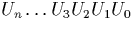Next: Signed binary fractions to Up: Signed Binary to Decimal Previous: Signed Binary to Decimal

### Signed binary integers to decimal

We can easily extract the integer part of a signed binary (mantissa, exponent) represented real by examining the exponent, and if it is positive, extracting that number of digits from the head of the stream. The remainder of the stream is the fractional part and is handled separately.

Converting the signed binary integer u to its decimal representationis performed as follows:

This differs slightly from the algorithm presented in  which uses the floor function instead of taking the integer part at each stage. The reason for this is that Knuth's algorithm only deals with unsigned digits. The `int' function behaves like the floor function for positive digits, but the ceiling function for negative digits (taking the integer obtained by rounding towards zero). This is the correct behaviour because converting negative digits is equivalent to negating these digits to make them positive and then negating the output digit at each stage.

This part of the algorithms requires `div' and `mod' operations to be performed on a finite signed binary list. This can be performed using a modification of the division of a stream by an integer algorithm described in section 4.3.3. It is changed so that the input list `div' the denominator is the output which would be returned from the original algorithm, and the `mod' portion is the remainder to be carried which is found when the end of the input list is reached.Next: Signed binary fractions to Up: Signed Binary to Decimal Previous: Signed Binary to Decimal
Martin Escardo
5/11/2000